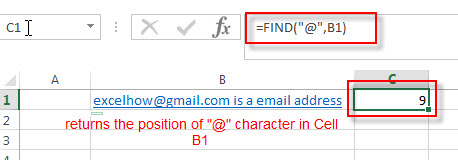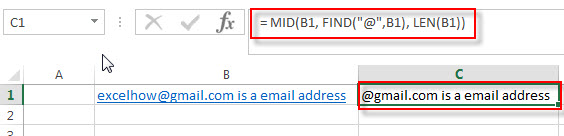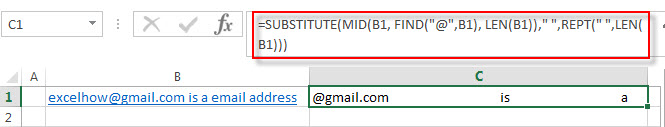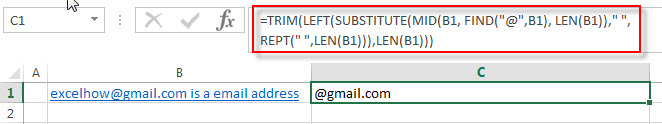# How to extract word that starting with a specific character

This post will guide you how to extract a word that starts with a specific character in excel.

## Extract word that starting with a specific character

For example, Assuming that you have a text string that contains email address in Cell B1, and if you want to extract word that begins with a specific character “@” sign, you can use a combination with the TRIM function, the LEFT function, the SUBSTITUTE function, the MID function, the FIND function, the LEN function and the REPT function to create an excel formula as follows:

`=TRIM(LEFT(SUBSTITUTE(MID(B1, FIND("@",B1), LEN(B1))," ",REPT(" ",LEN(B1))),LEN(B1)))`

Let’s see how this formula works:

=LEN(B1)

The LEN function returns the number of characters in a text string in Cell B1. The returned result goes into the MID function as its num_chars argument.

= FIND(“@”,B1)The FIND function returns the position of the first specific character “@” sign in Cell B1. It returns 9. It goes into the MID function as its start_num argument.

=MID(B1, FIND(“@”,B1), LEN(B1))The MID function extracts a substring at the starting position (returned by the FIND function) and num_chars value (returned by LEN function)

=REPT(” “,LEN(B1))

This formula repeats empty string a specified number of times returned by the LEN function. It will go into the SUBSTITUTE function as its new_text argument.

= SUBSTITUTE(MID(B1, FIND(“@”,B1), LEN(B1)),” “,REPT(” “,LEN(B1)))The SUBSTITUTE function will replace all empty string with another new text string returned by the REPT function from a text string returned by the MID function.  Then the returned value goes into the LEFT function as its Text argument.

= LEFT(SUBSTITUTE(MID(B1, FIND(“@”,B1), LEN(B1)),” “,REPT(” “,LEN(B1))),LEN(B1))The LEFT function extracts a specified number of the characters from a text string returned by the SUBSTITUTE function, starting from the leftmost character and the num_chars value is equal to the length of the string in Cell B1.

=TRIM()The TRIM function removes all spaces from text string returned by the LEFT function, just leave one space between words.

### Related Formulas

• Split Multiple Lines from a Cell into Rows
If you have multiple lines in a cell and each line is separated by line break character or press “alt + enter” key while you entering the text string into cells, and you need to extract the multiple lines into the separated rows or columns, you can use a combination with the TRIM function, the MID function, the SUBSTITUTE function, the REPT function, the LEN function to create a complex excel formula..…
• Extract word that containing a specific character
If you want to extract word that contains a hash character in a text string in Cell B1, you can use a combination of the TRIM function, the MID function, the SUBSTITUTE function, the REPT function, the FIND function to create an excel formula.…

### Related Functions

• Excel Substitute function
The Excel SUBSTITUTE function replaces a new text string for an old text string in a text string.The syntax of the SUBSTITUTE function is as below:= SUBSTITUTE  (text, old_text, new_text,[instance_num])….
• Excel TRIM function
The Excel TRIM function removes all spaces from text string except for single spaces between words.  You can use the TRIM function to remove extra spaces between words in a string.The syntax of the TRIM function is as below:= TRIM (text)….
• Excel LEFT function
The Excel LEFT function returns a substring (a specified number of the characters) from a text string, starting from the leftmost character.The syntax of the LEFT function is as below:= LEFT(text,[num_chars])….
• Excel MID function
The Excel MID function returns a substring from a text string at the position that you specify.The syntax of the MID function is as below:= MID (text, start_num, num_chars)….
• Excel FIND function
The Excel FIND function returns the position of the first text string (sub string) within another text string.The syntax of the FIND function is as below:= FIND(find_text, within_text,[start_num])…
• Excel LEN function
The Excel LEN function returns the length of a text string (the number of characters in a text string).The syntax of the LEN function is as below:= LEN(text)…
• Excel REPT function
The Excel REPT function repeats a text string a specified number of times.The syntax of the REPT function is as below:= REPT  (text, number_times)…
Related Posts

How to Remove All Extra Spaces and Keep Only One Between Words in Excel

Sometimes when copying something from other type files to excel, there might be two or more spaces display between words. Extra spaces between words are frequently to be seen in excel, and this behavior is very annoying. If we want ...

How to Remove Middle Name from Full Name in Excel

When we get a list of full names, we can remove the middle name for short in some cases. As the middle names are different among the full name list so we cannot replace them by space directly in excel. ...

How to Count Comma Separated Value in One Cell in Excel

This post will guide you how to count values in a single cell separated by commas with a formula in Excel. How do I count comma separated values in one cell in Excel 2013/2016. Is it possible to have a ...

How to Remove the First/Last Word from Text string in Cell

This post will guide you how to remove the first and the last word from a text string in cells in Excel. How do I use a formula to remove first and last word of a text string in Excel. ...

This post will guide you how to extract email address from a text string in Excel. How do I use a formula to extract email address in Excel. How to extract email address from text string with VBA Macro in ...

Remove Trailing Spaces from Cells in Excel

This post will guide you how to remove leading and trailing spaces in cells in Excel. How do I remove leading or trailing spaces in a range of cells with a formula in Excel. How to remove trailing spaces from ...

Convert Scientific Notation to Text or a Number in Excel

This post will guide you how to convert scientific notation to text or a number in Excel. How do I convert excel scientific to text with a formula in Excel. Convert Scientific Notation to Text or a Number in Excel ...

Reverse Concatenate Formula in Excel

This post will guide youw how to reverse the concatenate function in Excel. how do I split the text string into the muliptle cells with a formula in Excel. How to split text data of a cell to multiple cells ...

Remove Spaces from a String

This post will guide you how to remove extra spaces between words or text string from the specified range of cells in excel. How to delete all spaces from excel cells. For example, when you paste data from an external ...